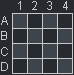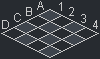# How do isometric coordinates work

There are a number of good tutorials on the Internet explaining the basic workings of the isometric projection related to game development in terms of tiles and offsets, while other tutorials give directly the coordinate transform formula without giving any explaination on how / why that formula works.

In this blog post I will try to explain how the isometric coordinate transform formula is obtained.

First of all, the world of the game can be represented by a simple 2D grid, for example a top down view might look like this:All the logic of the game will use this simple 2D grid, so let's call this the Grid coordinate system.

We want to display the world of the game in a projection like this:Let's call this projection the Screen coordinate system.

You may have seen some blog posts that convert from Grid coordinate system to Screen coordinate system in terms of tile position and offset inside the tile, but, as not every game is tile based, I prefer to convert absolute pixel values. Moreover, the conversion from absolute pixel values to tile position and offset inside the tile and vice versa is much simpler to do directly in the Grid coordinate system, instead of adding this extra complexity in the transforms.

Ok, so let's start the transform by rotating the view by $$\frac{\pi}{4}$$ radians (45°).

In order to rotate the coordinates, we will use the rotate transform matrix, that is defined as follows:

\begin{equation*} \begin{bmatrix} \cos{\alpha} & \sin{\alpha} & 0 \\ -\sin{\alpha} & cos{\alpha} & 0 \\ 0 & 0 & 1 \end{bmatrix} \end{equation*}

We know that both $$\cos{\frac{\pi}{4}}$$ and $$\sin{\frac{\pi}{4}}$$ equals to $$\frac{1}{\sqrt{2}}$$, so the matrix is as follows:

\begin{equation*} \begin{bmatrix} \frac{1}{\sqrt{2}} & \frac{1}{\sqrt{2}} & 0 \\ -\frac{1}{\sqrt{2}} & \frac{1}{\sqrt{2}} & 0 \\ 0 & 0 & 1 \end{bmatrix} \end{equation*}

So far, so good. Now we need the height to be half of the width, and in order to do so we will multiply the result by the scale transform matrix, that is defined as follows:

\begin{equation*} \begin{bmatrix} scale x & 0 & 0 \\ 0 & scale y & 0 \\ 0 & 0 & 1 \end{bmatrix} \end{equation*}

By setting $$scale y$$ to $$\frac{1}{2}$$, the multiplication will be:

\begin{equation*} \begin{bmatrix} \frac{1}{\sqrt{2}} & \frac{1}{\sqrt{2}} & 0 \\ -\frac{1}{\sqrt{2}} & \frac{1}{\sqrt{2}} & 0 \\ 0 & 0 & 1 \end{bmatrix} \times \begin{bmatrix} 1 & 0 & 0 \\ 0 & \frac{1}{2} & 0 \\ 0 & 0 & 1 \end{bmatrix} = \begin{bmatrix} \frac{1}{\sqrt{2}} & \frac{1}{2\sqrt{2}} & 0 \\ -\frac{1}{\sqrt{2}} & \frac{1}{2\sqrt{2}} & 0 \\ 0 & 0 & 1 \end{bmatrix} \end{equation*}

The resulting matrix could be good enough, but there is still this $$\frac{1}{\sqrt{2}}$$ everywhere that complicates the equation, so let's scale everything by $$\sqrt{2}$$, again by multiplying the result by the scale transform matrix:

\begin{equation*} \begin{bmatrix} \frac{1}{\sqrt{2}} & \frac{1}{2\sqrt{2}} & 0 \\ -\frac{1}{\sqrt{2}} & \frac{1}{2\sqrt{2}} & 0 \\ 0 & 0 & 1 \end{bmatrix} \times \begin{bmatrix} \sqrt{2} & 0 & 0 \\ 0 & \sqrt{2} & 0 \\ 0 & 0 & 1 \end{bmatrix} = \begin{bmatrix} 1 & \frac{1}{2} & 0 \\ -1 & \frac{1}{2} & 0 \\ 0 & 0 & 1 \end{bmatrix} \end{equation*}

That's it. By using this matrix, we can finally use this transform with our code:

var
screen_x = grid_x - grid_y,         // (1 * grid_x) + (-1 * grid_y) + (0 * 1)
screen_y = (grid_x + grid_y) / 2    // ((1 / 2) * grid_x) + ((1 / 2) * grid_y) + (0 * 1)
;


Of course, by using the inverse matrix we can convert from Screen coordinates to Grid coordinates. I will not demonstrate it, but the inverse of our matrix is:

\begin{equation*} \begin{bmatrix} \frac{1}{2} & -\frac{1}{2} & 0 \\ 1 & 1 & 0 \\ 0 & 0 & 1 \end{bmatrix} \end{equation*}

And the code for this transform will be:

var
grid_x = screen_y + (screen_x / 2), // ((1 / 2) * screen_x) + (1 * screen_y) + (0 * 1)
grid_y = screen_y - (screen_x / 2)  // (-(1 / 2) * screen_x) + (1 * screen_y) + (0 * 1)
;


That's all, folks. I hope that now it is clear how and why the coordinate conversions work, see you in the next blog post.